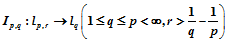# 无穷维恒等算子的伪宽度Pseudo n-Width of Infinite Dimension Identity Operator

• 全文下载: PDF(506KB)    PP.747-752   DOI: 10.12677/AAM.2019.84084
• 下载量: 158  浏览量: 229

In this paper, we study the pseudo width of infinite dimension identity operator, and obtain its asymptotic degree.

1. 引言及主要结果

$\left\{\mathrm{sgn}\left(F|{}_{I,y}\right):F|{}_{I,y}=\left({x}_{{i}_{1}}+{y}_{1},\cdots ,{x}_{{i}_{k}}+{y}_{k}\right),x=\left({x}_{1},\cdots ,{x}_{m}\right)\in F,{i}_{j}\in I,1\le j\le k\right\}$

${\rho }_{n}\left(W,Z\right)=\underset{{F}_{n}}{\mathrm{inf}}\underset{x\in W}{\mathrm{sup}}\underset{y\in {F}_{n}}{\mathrm{inf}}‖x-y‖$

$W$$Z$ 中的伪宽度，其中 ${F}_{n}$ 取遍 $Z$ 中的伪维数不超过 $n$ 的所有线性子空间。

${\rho }_{n}\left(T:X\to Y\right)={\rho }_{n}\left(T\left({B}_{z}\right),\stackrel{¯}{Y}\right)$

$1\le p\le \infty$ ，对任一实序列 $x={\left\{{x}_{n}\right\}}_{n=1}^{\infty }$ ，令

${‖x‖}_{{l}_{p}}=\left\{\begin{array}{cc}{\left(\underset{n=1}{\overset{\infty }{\sum }}{|{x}_{n}|}^{p}\right)}^{1/p},& 1\le p<\infty \\ \underset{n\ge 1}{\mathrm{sup}}|{x}_{n}|,& p=\infty \end{array}$

${l}_{p}$ 表示满足条件 ${‖x‖}_{{l}_{p}}<\infty$ 的实序列 $x$ 所构成的集合。众所周知， ${‖·‖}_{{l}_{p}}$${l}_{p}$ 上的一个范数，而且 ${l}_{p}$ 是一个Banach空间。易见， ${l}_{p}$ 空间具有如下性质：

1) ${l}_{p}\subset {l}_{q}\left(1\le p\le q\le \infty \right)$

2) ${l}_{p}\not\subset {l}_{q}\left(1\le p\le q\le \infty \right)$

${x}^{r}:={\left\{{n}^{r}{x}_{n}\right\}}_{n=1}^{\infty }$${‖x‖}_{{l}_{p,r}}={‖{x}^{\left(r\right)}‖}_{{l}_{p}}$

${l}_{p,r}:=\left\{x\in {l}_{p}|{‖x‖}_{{l}_{p,r}}<\infty \right\}$

$1\le q$r>\frac{1}{q}-\frac{1}{p}$ ，( $p=\infty$ 时，记 $\frac{1}{p}=0$ )，对任意的 $x=\left\{{x}_{n}\right\}\in {l}_{p,r}$ ，由Hölder不等式有

${‖x‖}_{{l}_{q}}\le \left\{\begin{array}{cc}{‖x‖}_{{l}_{p.r}}{\left(\underset{n=1}{\overset{\infty }{\sum }}{n}^{-\frac{pr}{p-q}}\right)}^{\frac{1}{q}-\frac{1}{p}}<\infty ,& 1\le q

${I}_{p,q}:{l}_{p,r}\to {l}_{q}$

$x↦x$

${l}_{p,r}$${l}_{q}$ 的有界线性算子。

${\rho }_{n}\left({I}_{p,q}:{l}_{p,r}\to {l}_{q}\right)\succ \prec {n}^{-\left(r-\frac{1}{q}+\frac{1}{p}\right)}$

2. 主要结果的证明

$1\le p\le \infty$$x=\left({x}_{1},\cdots ,{x}_{m}\right)\in {ℝ}^{m}$ 。令

${‖x‖}_{{l}_{p}^{m}}=\left\{\begin{array}{cc}{\left(\underset{n=1}{\overset{m}{\sum }}{|{x}_{n}|}^{p}\right)}^{1/p},& 1\le p<\infty ,\\ \underset{1\le n\le m}{\mathrm{max}}|{x}_{n}|,& p=\infty .\end{array}$

${‖·‖}_{{l}_{p}^{m}}$${ℝ}^{m}$ 上的范数。用 ${l}_{p}^{m}$ 表示 ${ℝ}^{m}$ 按照范数 ${‖·‖}_{{l}_{p}^{m}}$ 所构成的Banach空间。用 ${B}_{p}^{m}$ 表示 ${l}_{p}^{m}$ 中的单位球。易见 ${\left\{{{e}^{\prime }}_{n}\right\}}_{n=1}^{m}$${l}_{p}^{m}$ 的基，其中 ${{e}^{\prime }}_{n}=\left(0,\cdots ,0,1,0,\cdots 0\right)\in {ℝ}^{m}$ (第n个分量为1，其余分量为0)。

${\rho }_{n}\left({B}_{p}^{m},{l}_{q}^{m}\right)\approx {\left(m-n\right)}^{\frac{1}{q}-\frac{1}{p}}.$

$\forall k\in ℕ$ ，其中 $ℕ=\left\{1,2,\cdots \right\}$ ，记 ${S}_{k}=\left\{n\in N\left\{{2}^{k-1}\le n<{2}^{k}\right\}$ 。则对任意的 $k,{k}^{\prime }\in ℕ$ ，且 $k\ne {k}^{\prime }$${S}_{k}\cap {S}_{{k}^{\prime }}=\varnothing$$ℕ=\underset{k=1}{\overset{\infty }{\cup }}{S}_{k}$ 。用 ${m}_{k}$ 表示 ${S}_{k}$ 中元素的个数，则 ${m}_{k}=|{S}_{k}|={2}^{k-1}$

$k\in ℕ$ ，记 ${F}_{k}=\text{span}\left\{{e}_{n}|n\in {S}_{k}\right\}$ ，则 ${\mathrm{dim}}_{\rho }\left({F}_{k}\right)={m}_{k}={2}^{k-1}$ 。令

${I}_{k}:{F}_{k}\to {R}^{{m}_{k}}$

$x=\underset{n\in {S}_{k}}{\sum }{x}_{n}{e}_{n}↦\underset{j=1}{\overset{m}{\sum }}{x}_{{2}^{k-1}+j-1}{{e}^{\prime }}_{{2}^{k-1}+j-1}$

$\begin{array}{c}{‖x‖}_{{l}_{p,r}}={\left(\underset{n\in {S}_{k}}{\sum }{|{n}^{r}{x}_{n}|}^{p}\right)}^{1/p}\succ \prec {\left(\underset{n\in {S}_{k}}{\sum }{|{2}^{rk}{x}_{n}|}^{p}\right)}^{1/p}\\ ={2}^{rk}{\left(\underset{n\in {S}_{k}}{\sum }{|{x}_{n}|}^{p}\right)}^{1/p}={2}^{rk}{‖{I}_{k}x‖}_{{l}_{p}^{{m}_{k}}}\end{array}$ (2.1)

${‖x‖}_{{l}_{p}}={\left(\underset{n\in {S}_{k}}{\sum }{|{x}_{n}|}^{p}\right)}^{1/p}={‖{I}_{k}x‖}_{{l}_{p}^{{m}_{k}}}.$ (2.2)

${\rho }_{n}\left({B}_{p,r},{l}_{q}\right)=\underset{n=1}{\overset{\infty }{\sum }}{2}^{-rk}{\rho }_{{n}_{k}}\left({B}_{p}^{{m}_{k}},{l}_{q}^{{m}_{k}}\right).$

$1\ge {‖x‖}_{{l}_{p,r}}={2}^{rk}{‖{I}_{k}x‖}_{{l}_{p}^{{m}_{k}}}$

$\forall y\in {F}_{k}$ ，由(2.2)知

${‖y‖}_{{l}_{q}}={‖{I}_{k}y‖}_{{l}_{q}^{{m}_{k}}}$

${\rho }_{{n}_{k}}\left({B}_{p,r}\cap {F}_{k},{l}_{q}\cap {F}_{k}\right)={2}^{-rk}{\rho }_{{n}_{k}}\left({B}_{p}^{{m}_{k}},{l}_{q}^{{m}_{k}}\right)$

$\underset{x\in {B}_{p,r}\cap {F}_{k}}{\mathrm{sup}}\underset{y\in {M}_{k}}{\mathrm{inf}}{‖x-y‖}_{{l}_{q}}={2}^{-rk}{\rho }_{{n}_{k}}\left({B}_{p}^{{m}_{k}},{l}_{q}^{{m}_{k}}\right)$

$M=\underset{k=1}{\overset{\infty }{\sum }}{M}_{k}$ (直和)。则 $M$${l}_{q}$ 的线性子空间，且

${\mathrm{dim}}_{\rho }\left(M\right)\le \underset{k=1}{\overset{\infty }{\sum }}{\mathrm{dim}}_{\rho }\left({M}_{k}\right)\le \underset{k=1}{\overset{\infty }{\sum }}{n}_{k}\le n$

$\begin{array}{c}{\rho }_{n}\left({B}_{p,r},{l}_{q}\right)=\underset{x\in {B}_{p,r}}{\mathrm{sup}}\underset{y\in M}{\mathrm{inf}}{‖x-y‖}_{{l}_{q}}\\ \le \underset{k=1}{\overset{\infty }{\sum }}\underset{x\in {B}_{p,r}\cap {F}_{k}}{\mathrm{sup}}\underset{y\in {M}_{k}}{\mathrm{inf}}{‖x-y‖}_{{l}_{q}}\\ =\underset{k=1}{\overset{\infty }{\sum }}{2}^{-rk}{\rho }_{{n}_{k}}\left({B}_{p}^{{m}_{k}},{l}_{q}^{{m}_{k}}\right)\end{array}$

${\rho }_{n}\left({B}_{p,r},{l}_{q}\right)={2}^{-rk}{\rho }_{n}\left({B}_{p}^{{m}_{k}},{l}_{q}^{{m}_{k}}\right)$

$1\ge {‖x‖}_{{l}_{p}^{{m}_{k}}}\ge {2}^{rk}{‖{I}_{k}{}^{-1}x‖}_{{l}_{p,r}}$

$\forall y\in {l}_{q}^{{m}_{k}}$ ，则由(2.2)有

${‖y‖}_{{l}_{q}^{{m}_{k}}}={‖{I}_{k}{}^{-1}y‖}_{{l}_{q}}$

$\begin{array}{c}{\rho }_{n}\left({B}_{p,r},{l}_{q}\right)\ge {\rho }_{n}\left({B}_{p,r}\cap {F}_{k},{l}_{q}\cap {F}_{k}\right)\\ ={2}^{-rk}{\rho }_{n}\left({B}_{p}^{{m}_{k}},{l}_{q}^{{m}_{k}}\right)\end{array}$

$\forall k\in ℕ$ ，令 ${k}^{\prime }=\left[\mathrm{lg}n\right]$

${n}_{k}=\left\{\begin{array}{cc}{m}_{k},& 1\le k<{k}^{\prime }\\ {2}^{{k}^{\prime }}·{2}^{\beta \left({k}^{\prime }-k\right)},& k\ge {k}^{\prime }\end{array}$

$\begin{array}{c}{\rho }_{n}\left({I}_{p,q}:{l}_{p,r}\to {l}_{q}\right)=\underset{n=1}{\overset{\infty }{\sum }}{2}^{-rk}{\rho }_{{n}_{k}}\left({B}_{p}^{{m}_{k}},{l}_{q}^{{m}_{k}}\right)\\ =\underset{k={k}^{\prime }}{\overset{\infty }{\sum }}{2}^{-rk}{\rho }_{{n}_{k}}\left({B}_{p}^{{m}_{k}},{l}_{q}^{{m}_{k}}\right)=\underset{k={k}^{\prime }}{\overset{\infty }{\sum }}{2}^{-rk}·{2}^{\left(\frac{1}{q}-\frac{1}{p}\right)k}\\ =\underset{k={k}^{\prime }}{\overset{\infty }{\sum }}{2}^{-\left(r-\frac{1}{q}+\frac{1}{p}\right)k}={2}^{-\left(r-\frac{1}{q}+\frac{1}{p}\right)\right){k}^{\prime }}={n}^{-\left(r-\frac{1}{q}+\frac{1}{p}\right)}\end{array}$

$\begin{array}{c}{\rho }_{n}\left({I}_{p,q}:{l}_{p,r}\to {l}_{q}\right)={2}^{-rk}{\rho }_{n}\left({B}_{p}^{{m}_{k}},{l}_{q}^{{m}_{k}}\right)\\ ={2}^{-rk}\cdot {n}^{\frac{1}{q}-\frac{1}{p}}={n}^{-\left(r-\frac{1}{q}+\frac{1}{p}\right)}\end{array}$

  Traub, J.F. Wasilkowski, G.W. and Wozniakowski, H. (1988) Information-Based Complexity. Academic Press, Bos-ton.  Vapnik, V.N. and Chervonenkis, A.Ya. (1971) On the Uniform Convergence of Relative Frequencies of Events to Their Probabilities. Theory of Probability & Its Applications, 16, 264-280. https://doi.org/10.1137/1116025  Vapnik, V.N. and Cbervonenkis, A.Ya. (1981) Necessary and Sufficient Conditions for the Uniform Convergence of Means to Their Expectations. Theory of Probability & Its Applications, 26, 532-553. https://doi.org/10.1137/1126059  Pollard, D. (1989) Empiricai Processes: Theory and Applications. NSF-CBMS Regional Conference Sews in Probability and Statistics, Vol. 2, Institute of Mathmatical Statistics and American Statistical Association.  Haussler, D. (1992) Decision Theoretic Generalizations of the PAC Model for Neural Net and Other Learning Applications. Information and Computation, 100, 78-150. https://doi.org/10.1016/0890-5401(92)90010-D  Vapnik, V.N. (1982) Estimation of Dependences Based on Empirical Data. Springer. Berlin.  Maiorova, V. and Ratsaby, J. (1998) The Degree of Approximation of Sets in Euclidean Space Using Sets with Bounded Vapnik-Chervonenkis Dimension. Discrete Applied Mathematics, 86, 81-93. https://doi.org/10.1016/S0166-218X(98)00015-8  Chen, G.G., Fang, G.S. and Ruan, Y.L. (2007) Non-Linear Approximation of Functions with Mixed Smoothness by Sets of Finite Pseudo-Dimension. Acta Mathematica Sinica, English Series, 23, 671-676. https://doi.org/10.1007/s10114-005-0921-x